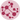# Custom NetworkX Graph Appearance

The previous post explains how to draw a basic network chart. In this post, we will have a look to the arguments that allows to custom the appearance of the chart.

The customisations are separated in 3 main categories: nodes, node labels and edges:

## Nodes

The `draw()` function of networkx library is used to draw the graph G with matplotlib. You can make customization to the nodes by passing these parameters to the function: node_size, node_color, node_shape, alpha, linewidths.

``````# libraries
import pandas as pd
import numpy as np
import networkx as nx
import matplotlib.pyplot as plt

# Build a dataframe with your connections
df = pd.DataFrame({ 'from':['A', 'B', 'C','A'], 'to':['D', 'A', 'E','C']})

G=nx.from_pandas_edgelist(df, 'from', 'to')

# Graph with Custom nodes:
nx.draw(G, with_labels=True, node_size=1500, node_color="skyblue", node_shape="s", alpha=0.5, linewidths=40)
plt.show()``````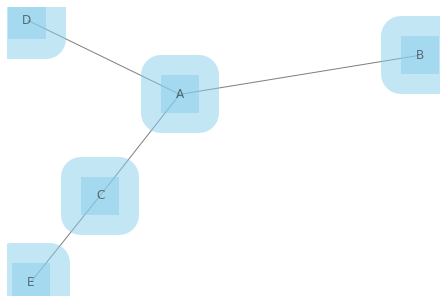## Labels

You can custom the labels of nodes by passing font_size, font_color and font_weight parameters to the function.

``````# libraries
import pandas as pd
import numpy as np
import networkx as nx
import matplotlib.pyplot as plt

# Build a dataframe with your connections
df = pd.DataFrame({ 'from':['A', 'B', 'C','A'], 'to':['D', 'A', 'E','C']})

G=nx.from_pandas_edgelist(df, 'from', 'to')

# Custom the labels:
nx.draw(G, with_labels=True, node_size=1500, font_size=25, font_color="yellow", font_weight="bold")
plt.show()``````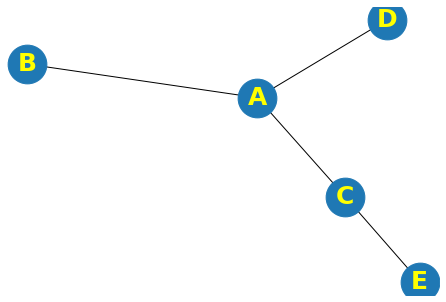## Edges

You can custom the edges by passing width, edge_color and style parameters to the function.

``````# libraries
import pandas as pd
import numpy as np
import networkx as nx
import matplotlib.pyplot as plt

# Build a dataframe with your connections
df = pd.DataFrame({ 'from':['A', 'B', 'C','A'], 'to':['D', 'A', 'E','C']})

G=nx.from_pandas_edgelist(df, 'from', 'to')

# Chart with Custom edges:
nx.draw(G, with_labels=True, width=5, edge_color="skyblue", style="solid")``````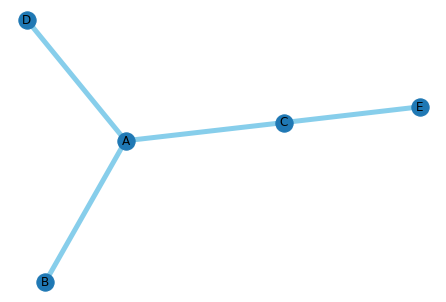## All

We can create a fancy network chart using all parameters described above:

``````# libraries
import pandas as pd
import numpy as np
import networkx as nx
import matplotlib.pyplot as plt

# Build a dataframe with your connections
df = pd.DataFrame({ 'from':['A', 'B', 'C','A'], 'to':['D', 'A', 'E','C']})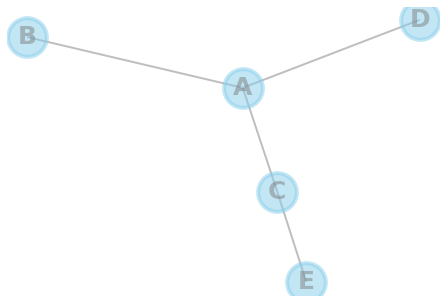👋 This document is a work by Yan Holtz. Any feedback is highly encouraged. You can fill an issue on Github, drop me a message onTwitter, or send an email pasting `yan.holtz.data` with `gmail.com`.